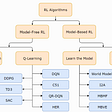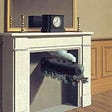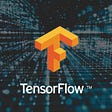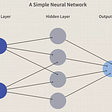# Multiclass Classification Using TensorFlow

In the previous article, I discussed building a linear regression model using Tensorflow. In this article, I will try to solve a multiclass classification problem using Tensorflow.

I have used the MNIST-digit recognizer dataset here. Please note that even though a Convolutional Neural Network might have worked better for this problem as this is an image recognition problem, but I have used a generic neural network as I wanted to showcase solving a classification problem using Neural Networks.

# Data Extraction and Exploration

The dataset consists of 784 pixel columns, where each row represents a 28 x 28 image flattened out into a row vector , and a label column, with the image labels given by the digits the image represent, from 0–9. This makes it a 10-class classification problem.

• Separate the features and labels into X_train and y_train
• Visualise some random images along with their labels

## Data Transformation

• Standardisation

For a neural network, it always helps to normalize the input data, so that the input values are between 0 and 1. For image input data, where the features are in the form of pixels, a quick way to standardize the data is to divide the feature vectors by 255, as the maximum pixel values normally in the range from 0–255.

• Train and Validation split

We already have the train and test data given to us. Lets further split the train data into train and validation data.

## Baseline Model

Start by building a baseline Tensorflow Sequential Model.

Evaluate on validation data

Plot the loss and accuracy curves

We can see that model is performing a lot better on training data than test data. In other words, the model is overfitting. To address this in a neural network, we can try the following among other things :

We start with the first option, by reducing the number of neurons in the hidden layers and build model 2 :

The performance has not improved. So I decide to find an optimal learning rate using Tensorflow’s learning rate callback

Define the learning rate scheduler callback

The way we have defined the learning rate callback above, ensures that the learning rate slowly increases with increasing epochs, starting from a value of 1e-4.

Fit the model including the callback:

Plot the learning rate versus loss curve to find the best learning rate:

From the curve we can see that when learning rate is about 0.005, the loss is still decreasing but not flattened out.

So we take 0.005 as our learning rate and retrain the model

Evaluate the model:

Plot the loss curves:

We see that with the new learning rate, the model is performing quite well, with a validation accuracy of about 94%. This is pretty impressive for a multi-class image classification problem, using a 3 layered neural network, without using a convolutional layer.

--

--

--

## More from Prakhar S

Data Scientist, Kode Tiger

Love podcasts or audiobooks? Learn on the go with our new app.

## Role of Mathematics in Image Processing.## Choosing the Right Business Problems for ML## Overview of explainable AI methods in NLP## A light timeline into computer vision. Part 1. Starting with machine learning.## Beginner to Advance: Having fun with Reinforcement learning #2## Churn Prediction using Neural Networks and ML models## The NLP Cypher | 02.21.21## Must Read Papers on Deep Residual Networks part1## Prakhar S

Data Scientist, Kode Tiger

## Linear Regression using Tensorflow## Vegetable Image Classification using CNN## Image Classification using Convoluted Neural Networks (Keras) — Deep Learning Algorithm## Semi-Supervised Learning | Pseudo Labeling Custom Dataset with YOLOv4